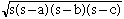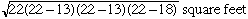What is the surface area of a triangle 13 ft x 13 ft x 18 ft Hi, There is this wonderful expression called Heron's formula that says If the sides of the triangle are a, b, c, and the semiperimeter is s (s = (a+b+c)/2), then the area is. When the sides are of length 13, 13 and 18 feet, s = 22 feet and thus the area isPenny Go to Math Central